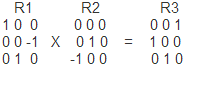# cv::Rodrigues problem

I have two rotation matricesR1 and R2 correspond the Euler angles: (90, 0 , 0) and (0, 0, 90)

as a result, I expect to get a turn like this 90, 0 , 90

But cv::Rodrigues returns this angles for R3: (69, 69, 69).

What I'm doing wrong

edit retag close merge delete

Sort by » oldest newest most voted

Just a google gave me that cv::Rodrigues does actually not at all what you want: http://docs.opencv.org/modules/calib3d/doc/camera_calibration_and_3d_reconstruction.html?highlight=camera%20calibration#rodrigues

You just want to combine two rotation matrices, thats something different right? Rodrigues outputs a vector, not a matrix...

more

In three-dimensional space, every rotation can be interpreted as a rotation by a given angle about a single fixed axis of rotation (see Eules Angles), and hence it can be simply described by an angle and a vector with three entries. However, it can also be represented by the nine entries of a rotation matrix with three rows and three columns. (WIKIPEDIA)

Rodrigues converts the Rotation Matrix to Eules Angles as i understand

In documentation Rodrigues Converts a rotation matrix to a rotation vector or (!!!)vice versa.

more

1

The ansver is simple: Rotation vector - is not an Euler Angles.

Official site

GitHub

Wiki

Documentation